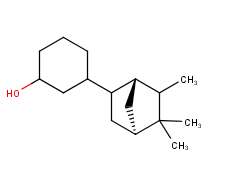1 Follower
0 Following
young

#### LocationCN

2
1
1

Dec
Jan
Feb
Mar
Apr
May
Jun
Jul
Aug
Sep
Oct
Nov
Dec
Mon
Wed
Fri

#### Challenges Entered

Predicting smell of molecular compounds

#### Latest submissions

 graded 93234 Tue, 27 Oct 2020 11:24:20 graded 93232 Tue, 27 Oct 2020 11:10:49 graded 93215 Tue, 27 Oct 2020 10:41:43

5 PROBLEMS 3 WEEKS. CAN YOU SOLVE THEM ALL?

#### Latest submissions

No submissions made in this challenge.
Participant Ratingcontrebande 0
Participant Rating

#### Learning to Smell### Multiple molecule in some SMILES

Another issue in the training data was the stereo bond. For example, #1015 OC1CCCC(C1)C1C[C@H]2C[C@H]1C(C2(C)C)C , the molecule looks like.
I don’t think these two stereo bond in the ring can be connected to one atom.### Multiple molecule in some SMILES

‘.’ in SMILES should mean two seperate molecule, here in the training set, some of the rows contains ‘.’ . Some of them should be salt, but some are just two molecules, for example 498 COCC1CC=C2C(C1)C(C)CC2(C)C.COCC1CC=C2C(C)CC(C)(C)C2C1.

Here is the full list:

70 CC(C(=O)[O-])O.[Na+]
90 CCCCc1nc(C)cnc1C.CCCCc1ncc(nc1C)C
142 CC1CCc2c(C1)occ2C.CC1CCC(C(C1)OC(=O)C)C(C)C.CC1CCC(=C(C)C)C(=O)C1.CC1CCC(C(=O)C1)C(C)C.CC1CCC(C(C1)O)C(C)C.CC1CCC2(CC1)OCC2C
248 OC1C[C@H]2C([C@]1(C)CC2)(C)C.C=CC(CCC=C(C)C)C.C=CCc1ccc(c(c1)OC)OC.OC/C=C(\CCC=C(C)C)/C.O=C/C=C(\CCC=C(C)C)/C
265 [NH4+].[NH4+].[S-2]
305 OCCOc1ccc(cc1N)N.Cl.Cl
454 C(C[C@@H](C(=O)O)N)CN.Cl
462 CC(CCCC(C)(C)O)CC=O.Cc1c[nH]c2ccccc12
498 COCC1CC=C2C(C1)C(C)CC2(C)C.COCC1CC=C2C(C)CC(C)(C)C2C1
507 CC(=O)O.OCCC=C(CCC=C(C)C)C
584 CCCCCCC(CCOC(=O)C)OC(=O)C.CCCCCCC(CCO)O
648 C=C1CC[C@H]2C[C@H]1C2(C)C.Cc1ccc(cc1)C(C)C.O=CC1=CCC(=CC1)C(C)C.O=Cc1ccc(cc1)C(C)C
994 CC(=O)OC/C(=C\CC[C@]1(C)C2C[C@@H]3C1(C)C3C2)/C.CC(=O)OC/C(=C\CC[C@]1(C)[C@H]2CC[C@@H](C1=C)C2)/C
1031 CC1=C(CCO1)[S-].CC(=O)O
1066 CCO[C@H]1[C@@H]([C@H]([C@@H]([C@@H](CO)O1)O)O)O.COc1cc(ccc1O)C=O
1288 C=C1[C@H]2CC[C@H]3[C@]1(C)CCCC([C@H]23)(C)C.FB(F)F.OC=O
1293 OCC(O)C.CCCCCCCCCCCCCCCCCC(=O)O
1542 CCCCCCCOP(=S)(OCCCCCCC)[S-].CCCCCCCOP(=S)(OCCCCCCC)[S-].[Zn+2]
1574 CCC(C)C(=O)C(=O)[O-].[Na+]
1611 CCC(=O)CCC1C(=CCCC1(C)C)C.C#CCO
1646 CCCCCCCCCCC=O.CCCCCCCCC(C)C=O
1681 O=C1C2(C)CCC(C1(C)C)C2.COc1ccc(cc1)CC=C.C/C=C/c1ccc(cc1)OC
1801 C/C(=C/CC[C@]1(C)C2C[C@H]3C(C2)C13C)/COC(=O)C.C/C(=C/CC[C@@]1(C)C(=C)[C@@H]2CC[C@H]1C2)/COC(=O)C
1915 N.O
1995 C[C@]1(O)CC[C@@H](CC1)C(O)(C)C.O
2087 COC(=O)[C@@H](Cc1cnc[nH]1)N.Cl
2149 CCCCCCCCCCCCC(S(=O)(=O)[O-])C(=O)OCC(CCCC)CC.[Na+]
2318 Cn1cnc2c1c(=O)n(C)c(=O)n2C.Cn1cnc2c1c(=O)[nH]c(=O)n2C.Oc1cc2OC(c3ccc(c(c3)O)O)C(Cc2c(c1)O)O
2389 CCCCCCCCCCCCOS(=O)(=O)[O-].[Na+]
2493 CCCCCCC(OC(=O)C)CCOC(=O)C.CCCCCCC(CCO)O
2540 C[S+](C)CC[C@@H](C(=O)[O-])N.Cl
2587 CC(C)CC(=O)C(=O)[O-].[Na+]
2820 CC1CCC2C(C)(C)C3CC12CC(=O)C3C.CC1C2CC23C(CCCC3(CC1=O)C)(C)C
2880 CC(=O)CCC/C=C/C=C/C=C.CC(=O)CCC/C=C\C=C\C=C.CCC(=O)CC/C=C/C=C/C=C.CCC(=O)CC/C=C\C=C\C=C.CCCC(=O)C/C=C/C=C/C=C.CCCC(=O)C/C=C\C=C\C=C
2888 C=C1CC[C@H]2C[C@@H]1C2(C)C.Cc1ccc(cc1)C(C)C.O=CC1=CCC(=CC1)C(C)C.O=Cc1ccc(cc1)C(C)C
2892 C/C/1=C\CCC(=C2/C(=C\C1)/CC2)C.CC(=CCC1=C(O)C(C(=O)C(=C1O)C(=O)CC(C)C)(CC=C(C)C)CC=C(C)C)C.CC(=CCC1=C(O)C(C(=O)C(=C1O)C(=C)CC(C)C)(O)CC=C(C)C)C
2899 COc1ccc(cc1)C(=O)OCC(=O)[O-].[Na+]
3005 C/C=C/c1ccccc1.C=CCc1ccc(c(c1)OC)O
3109 CCCCCCCCCCCCCCCCCC(=O)O.CC(CO)O
3144 Cc1ccccc1C(O)O.C(C(CO)O)O
3190 CCc1cnc(C)cn1.CCc1cncc(C)n1
3216 CCC(=O)CCC1C(=CCCC1(C)C)C.OCC#C
3254 O=CC1C(C)CC(=CC1C)C.O=CC1CC(=CC(C1C)C)C
3263 OC(c1ccccc1C)O.OCC(CO)O
3347 COc1cc(ccc1[O-])/C=C/C(=O)O.[Na+]
3602 CCc1c(C)nc(C)cn1.CCc1c(C)ncc(C)n1
3671 NCCSS(=O)(=O)[O-].[Na+]
4008 CC[C@@]1(O)C(=O)OCc2c1cc1c3nc4ccc(c(c4cc3Cn1c2=O)CN(C)C)O.Cl
4014 CC(C)CC(=O)O.N
4119 CC(C(=O)O)C.CCOc1cc(C=O)ccc1[O-]
4210 CC(C)c1cnc(cn1)OC.CC(C)c1cncc(n1)OC.CC(C)c1c(nccn1)OC
4250 OC1CC(OC1COP(=O)(OP(=O)(OP(=O)(O)[O-])[O-])O)n1cnc2c1ncnc2N.[Na+].[Na+]### What is the meaning of top2 in the new leaderboard?

Then how the metric would be in the final round to determine the winner? Is it still the top5 value or the mean of top2 and top5 or anything others?### What is the meaning of top2 in the new leaderboard?

Today I found that the leaderboard have updated, what is top2 mean? Is it the first and second setence in our 5 sentence submission?Will the entrance of round 2 and round 3 be restricted? For example, only 10% on top of the submitters are qulified to enter round 2 and round 3.### Can we use third party data?

I’ve notice that we can actually find some data from internet. eg. data from http://www.thegoodscentscompany.com/### What is the cause of ambiguity of structure and odor label

Question1: The essence about ordor comes from molecule and protein interaction, it’s very complicated. Think about the key and lock, small change in key would make it not working.
Question2: A paper about it. Is It Possible to Predict the Odor of a Molecule on the Basis of its Structure?, I did not find an answer yet.### Number of submissions

It’s 5 submissions per 24h.Over 3 years ago

How would the the score be calculated when the ground truth had less than 3 descriptions or more than 3 descriptions?
Should our prediction always has 3 kinds of smell in each sentence?### Interpretation of repeated "sentence" values

Over 3 years ago

Is that mean that, for C=CCS, if the prediction was ‘alliaceous, cooked, roasted’, it would get higher score compared to ‘cooked, roasted, alliaceous’?
As far as I know, Jaccard Index is not order related.

young has not provided any information yet.